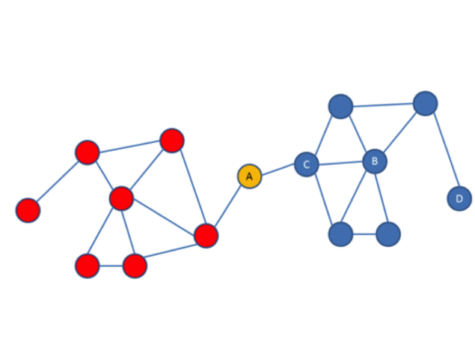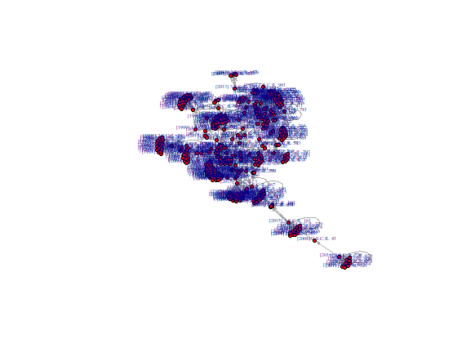# Lesson 4

### Introduction

Networks are a powerful tool that help us better understand the law.

First, the law itself is full of networks: statutes that refer to each other, cases that cite prior cases, contracts that connect contractors, lawyers that work together and so forth. Network analysis helps understand the law’s network structures and this structure’s effects.

Second, network analysis comes with an entire toolkit to investigate network structures. Many of these measures have a foundation in human intuition. Consider the simple network below.• – Who is the most powerful actor in that network?
• – Who would you like to be friends with?
• – What new link is likely to be created next?
• – What is the likely social role of A or B?
• – What happens if A leaves the network?
• – What real life networks could this image represent?

Network analysis provides a framework to turn these intuitions into metrics. A node that has many connections, like node B, has a high “degree centrality” in the network. Node A, in contrast, has a high “betweenness” score, because it connects different groups of the same network.

Third, network analysis is scalable. That means it allows researchers to quickly analyze large amounts of information. What is the most important decision made by the Supreme Court of Canada in the last 20 years? A network analysis of citations by the Supreme Court of Canada’s judgements can provide an answer in seconds without manually reviewing thousands of cases.

Finally, network analysis provides an appealing way to visualize legal information.

###### What we will do in this lesson

We will primarily work on one aspect of legal network analysis: the citation networks of courts.

• 1. Using regexes to find citations
• 2. Creating a citation list
• 3. Finding most cited cases
• 4. Visualizing networks
• 5. Network measures

### R Script

##### Using Regexes to Find Court Citations
Now, let's pick up where we left off in Lesson 3 and use regex to extract the citations from court decisions. Here, we will once again use Supreme Court of Canada data.
```# Activate package
```
```
```
``` # Load the Supreme Court of Canada example data into a folder on your hard drive. Write the path to that target folder.

```
```folder <- "~/Google Drive/Teaching/Canada/Legal Data Science/2019/Data/Supreme Court Cases/*"
```
```
# Upload the texts from that target folder.
```
```
```
We now want to identify the citations of other Supreme Court decisions found in these decisions. How can we do that? Every Supreme Court of Canada decision has a unique reference based on its Supreme Court Report. These references use the following format: " 1 S.C.R. 467." We can use the unique case reference to build our regex. The regex would thus be "\\[\\d+]\\s\\d+\\sS.C.R.\\s\\d+"
```
pattern <- "\\[\\d+]\\s\\d+\\sS.C.R.\\s\\d+"

```
##### Creating a Citation List

We now want to extract instances where another Supreme Court decision is cited within our text. For that, we need to loop through all our texts and extract the citation.

As output, we want to have a dataframe with 2 columns. Column 1 will contain the case that contains the citation. Column 2 will contain the case that was cited.

Since we have multiple cases, we need to do a for-loop. We start with an empty shell that we will populate with citations.

``` # Here our dataframe has 2 columns for citing and cited cases.

```
```all_citations <- data.frame(matrix(ncol = 2, nrow = 0))
```

Our loop will do two things. First, it will extract the cases that were cited. Second, we will add the case that had the citation in its text.

```
for (row in 1:nrow(scc)) {
```
```# we first focus on the text of each decision

```
```
case_text <- scc[row, "text"] # we first focus on the text of each decision
```
```# and search for our pattern in it

```
```citation_matcher <- gregexpr("\\[\\d+]\\s\\d+\\sS.C.R.\\s\\d+", case_text)
```
```# we then save all the cases cited in that decision

```
```citations <- regmatches(case_text, citation_matcher)[]
```
```# second we focus on the name of the citing case

```
```
case_name <- scc[row, "doc_id"]
```
```# and repeat so that every cited case can be accompanied by the name of the decision citing it

```
```case_name <- strrep(case_name,length(citations))
```

```
case_name <- strsplit(case_name,".txt")[]
```
```# then we match cited and citing cases

```
```
citation_list <- cbind(case_name, citations)
```
```# and save all these references in a dataframe

```
```all_citations <- rbind(all_citations,citation_list)
}

```

Here, we see that a case will often cite another case multiple times. It is possible to incoporate this information into an analysis by using it to determine the weight of various network ties. Ties would be weighted more heavily when one case cites another multiple times.

Today, however, we want to simplify our data so that cases are connected by a tie irrespective of the number of times a citation occurs.

```
# We do that by eliminating duplicates.

```
```
all_citations <- unique(all_citations)
```

##### Finding Most Citing and Cited Cases

We can now use our citation list to find the most-citing and most-cited cases. To accomplish this, we use the function table(). This function provides us with frequencies of the values in our dataframe. We also sort our dataframe to make it easier to identify the most cited or citing case.

```# Based on our citation list, we can find the most citing and most cited cases in our list.

```
```
most_citing <- as.data.frame(sort((table(all_citations\$case_name)), decreasing = TRUE))
```

```most_citing
```
``` ##
Var1 Freq1     1 S.C.R. 61   532    1 S.C.R. 623   423      1 S.C.R. 3   374    2 S.C.R. 398   355    1 S.C.R. 467   316    3 S.C.R. 511   317   3 S.C.R. 1101   278    1 S.C.R. 613   249    2 S.C.R. 167   2310   1 S.C.R. 130   2311  3 S.C.R. 1089   2212    1 S.C.R. 99   2013   2 S.C.R. 227   1914   1 S.C.R. 161   1715   2 S.C.R. 256   1516      1 SCR 704   1217   1 S.C.R. 180   1118  1 S.C.R. 1069   1119   2 S.C.R. 720   1020   2 S.C.R. 447    921   2 S.C.R. 548    922  1 S.C.R. 1099    923  3 S.C.R. 1053    624   1 S.C.R. 575    525     2 S.C.R. 3    3

```

Hence, the most citing case in our network is Quebec (Attorney General) v. A, 2013 SCC 5,  1 S.C.R. 61.

We can do the same thing with the most-cited cases. This list will be considerably longer, so we restrict ourselves to the 50 most cited cases.

```
most_cited <- as.data.frame(sort((table(all_citations\$citations)), decreasing = TRUE))
```

```most_cited[1:50]
```
``` ##                    Var1 Freq1     1 S.C.R. 295    82     3 S.C.R. 511    83     1 S.C.R. 103    64     2 S.C.R. 567    55     3 S.C.R. 199    56    1 S.C.R. 1075    57    3 S.C.R. 1010    58     2 S.C.R. 483    49     2 S.C.R. 507    410    3 S.C.R. 388    411    3 S.C.R. 103    412    1 S.C.R. 623    413     1 S.C.R. 27    414   3 S.C.R. 1101    415    1 S.C.R. 433    416    2 S.C.R. 257    417    2 S.C.R. 713    318    1 S.C.R. 396    319    3 S.C.R. 555    320    2 S.C.R. 203    321    2 S.C.R. 307    322    3 S.C.R. 458    323     1 S.C.R. 61    324    2 S.C.R. 335    325    2 S.C.R. 217    326    2 S.C.R. 235    327    2 S.C.R. 236    328    3 S.C.R. 550    329    3 S.C.R. 571    330     1 S.C.R. 44    331    3 S.C.R. 134    332    2 S.C.R. 145    333    1 S.C.R. 688    334    2 S.C.R. 650    335    3 S.C.R. 698    336    1 S.C.R. 927    237    3 S.C.R. 697    238    2 S.C.R. 513    239    1 S.C.R. 825    240    2 S.C.R. 551    241    1 S.C.R. 256    242    2 S.C.R. 610    243    1 S.C.R. 190    244    1 S.C.R. 160    245    3 S.C.R. 471    246    3 S.C.R. 654    247    1 S.C.R. 467    248     1 S.C.R. 30    249   1 S.C.R. 1296    250    1 S.C.R. 143    2
```

Hence, the most cited case in our network is R v Big M Drug Mart Ltd.,  1 S.C.R. 295 and Haida Nation v British Columbia,  3 S.C.R. 511. The former is landmark Charter case and the latter is a leading case on the Crown's duty to consult Aboriginal groups.

Since we worked with a small sample of 25 decisions for this analysis, we should not make too much of these findings. If a similar analysis were applied to all Canadian Supreme Court cases, we would fine meaningful patterns about what cases are the most-cited and most-citing.

##### Visualizing Networks

We can next proceed to visiualizing this network in R.

A couple of packages in R deal with network analysis . The most popular of them is igraph.

```library(igraph)
```
```
# The input is our 2-column matrix with the citation list.

```
```citation_network <- graph_from_edgelist(as.matrix(all_citations), directed = TRUE)
```

We can then use the plot() function to visualize the network.

```
plot(citation_network, layout=layout_with_fr, vertex.size=4,
vertex.label.dist=0.5, vertex.color="red", edge.arrow.size=0.2, vertex.label.cex=0.4)

```
``` ##```

While igraph is highly customizable, I personally prefer to work with self-standing network visualization tools. One of them is called "visone". It can be downloaded free of charge online: http://visone.info.

##### Network Measures
The igraph package can also calculate network measures. Several network measures exist that correspond to different node characteristics. Here we want to focus on two of them that are particularly useful for legal citation analysis:
1. Authority scores measure the importance of a node in a network based on the inward ties it attracts. In a legal citation network, this indicate that the case is a particularly important precedent.
2. Hub scores measure the importance of a node in a network based on the outward ties it sends. In a legal citation network, this would correspond to a decision that cites all relevant authorities.
We start by calculating the authority scores of our nodes to find the most important precedents.
```authority_score_network <- authority_score(citation_network, scale = TRUE, weights = NULL, options = arpack_defaults)
```
```citation_authority <- as.data.frame(sort(authority_score_network\$vector, decreasing=TRUE))
```
Let's compare the raw scores based on the number of citations with the authority scores. You will notice that the ranking is a bit different since authority scores weigh the importance of inward citations based on the importance of the citing node.
```head(most_cited)
```
` ## Var1 Freq`
1  1 S.C.R. 295 8
2  3 S.C.R. 511 8
3  1 S.C.R. 103 6
4  2 S.C.R. 567 5
5  3 S.C.R. 199 5
6  1 S.C.R. 1075 5
```

```
```

```
` ## sort(authority_score_network\$vector, decreasing = TRUE)`
 1 S.C.R. 295 1.0000000
 1 S.C.R. 103 0.8237840
 2 S.C.R. 567 0.7722282
 3 S.C.R. 199 0.7282003
 2 S.C.R. 713 0.5659069
 1 S.C.R. 396 0.5063234
```

```
We then turn to hub scores to find decision that well describes the status of the law by citing all relevant authorities.
```hub_score_network <- hub_score(citation_network, scale = TRUE, weights = NULL, options = arpack_defaults)
```
```citation_hub <- as.data.frame(sort(hub_score_network\$vector, decreasing=TRUE))

```
Let's again compare the raw scores based on the number of outward citations with the hub scores. You notice again that the ranking is a bit different, because hub scores weigh the importance of outward citations based on the importance of the cited node.
```head(most_citing)
```
` ## Var1 Freq`
1  1 S.C.R. 61 53
2  1 S.C.R. 623 42
3  1 S.C.R. 3 37
4  2 S.C.R. 398 35
5  1 S.C.R. 467 31
6  3 S.C.R. 511 31
```

```
```head(citation_hub)
```
` ## sort(hub_score_network\$vector, decreasing = TRUE)`
 1 S.C.R. 61 1.0000000
 1 S.C.R. 3 0.4699375
 1 S.C.R. 467 0.4162001
 2 S.C.R. 398 0.3568186
 1 S.C.R. 613 0.3111047
 3 S.C.R. 1101 0.2818147
```

```
Which network metrics best describe the varying characteristics of a legal case is an area of ongoing research. Generally, however, different network measures correspond to different attributes of a case. As a result, selecting an appropriate network measure depends on what you are trying to assess.